# Stock forms of Components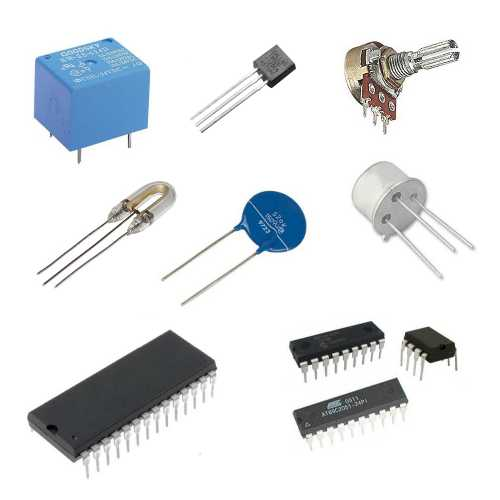## 1 Introduction

### Tolerances, Ratings and Values

• Electronic components are not available in any value, they are sold in different vlaue ranges.
• The most common type of resistor values is the 'E12' standard.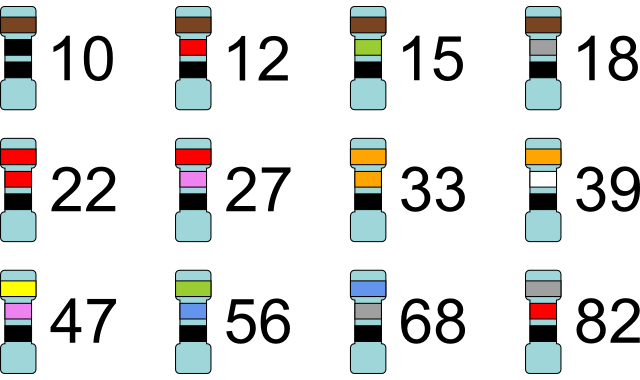• for each value in the E12 series, we simply add a zero onto each number to get the next range up and then another zero and so on.
• For example: 12 becomes 120 and then 1200 and then 12 000 ohms.
• All resistors will be produced with a tolerance, the most common being +/- 5%. This is due to manufacturing processes not being perfect.
• You can get resistors with a tolerance as low as +/- 1%, but these will cost more due to the extra care needed to manufacture these.
• Other values which are important are the maximum input voltage and current rating, for example, all products that are plugged into the mains supply must be rated at 240V.
• If the rating is not high enough, this could cause serious damage to the product as well a being a serious fire hazard.
• This would apply to battery operated devices as well.

### Through-hole compnents

• THese are the components you are most aware of, they are components with wire legs that are bent into place and then pushed through a hole in the PCB and then soldered on the opposite side onto copper tracks.
• These components are very useful for hobbyists and the prototyping and testing of PCB designs.
• They are mainly used for small scale manufacture.

### Surface mounted components

• Surface-mount technology (SMT) is a way of fixing components onto the PCB instead of through holes.
• There are copper tracks on top of the board and all the components are laid on the PCB in place.
• Each component has solder in place on itself. The PCB with the components is then passed through and oven which melt the solder and solders the components in place.
• This process is used in high speed manufacture as robots are able to pick and place the components very quickly.

### Sizes

• It is important to know what units are used in calculations.
• Each unit is given a symbol that goes after the number.
• The unit is often prefixed with a multiplier, see table below.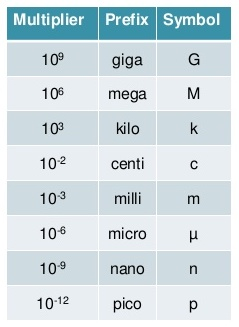### Unit of current

• Cureent is the measure of the flow of electric charge. It is the measurement of the number of electrons passing a point in the conductor per second.
• Current is measured in amperes (amps).
• The symbol for current is 'A'

### Unit of resistance

• The is the measurement of how easy or difficult it is for electrons to move through a conductor.
• Resistance is measured in ohms.
• THe symbol for resistance is the greek symbol for omega 'Ω'

### Unit of potential difference (voltage)

• Potential difference (voltage) is the amount of force available to force electrons to move (electricity to flow).
• PD is measured in voltage.
• The symbol for PD is a capital 'V'.

## 2 Ohm's Law

### Tolerances, Ratings and Values

• Ohm's law is the relationship between current, voltage and resistance. It states:
• The potential difference (voltage) across an ideal conductor is proportional to the current through it. The constant of proportionality is called the "resistance", R. Ohm's Law is given by: V = I R where V is the potential difference between two points which include a resistance R.
• To simplify the law:
1. When the voltage increases, the current increases. Because there is less force available.
2. When the resistance is increases, the current decreases as it is more difficult for current to flow with more resistance.
• Ohm's law calculations are very important in electronics.
• V = I X R
• I = V / R
• R = V / I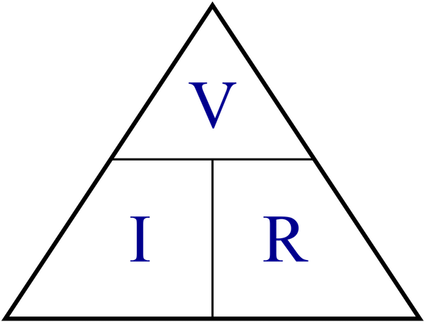### Resistors in series

• When resistors are connected one after another, then they are connected in series.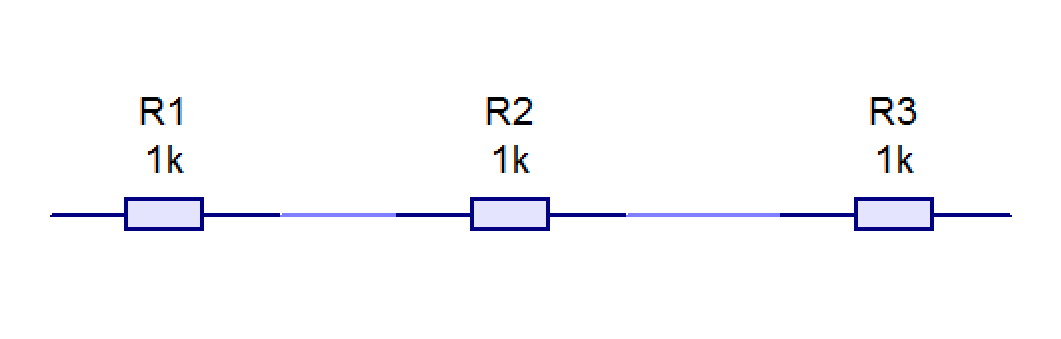• To calculate the total resistance, we simply add the values together.
• Rtotal = R1 + R2 + R3

### Resistors in parallel

• Resistors connected on top of one another are connected in parallel.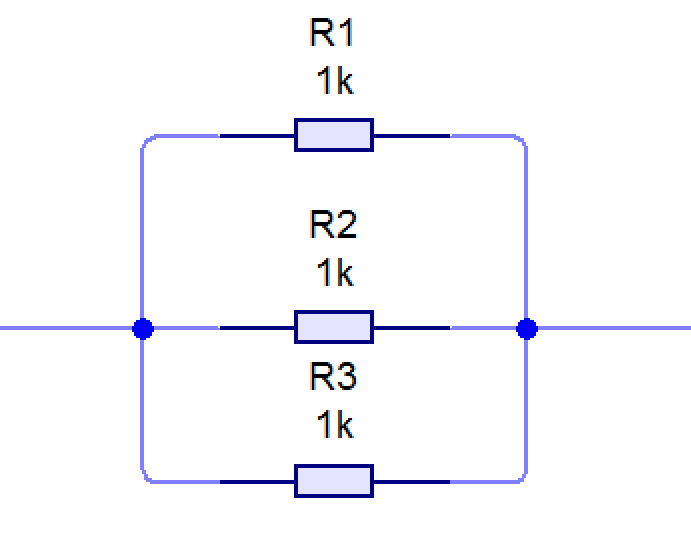• To calculate the total resistance in parallel, is a little more complicated than in series.
• You need to calculate the 'reciprocals' of all the resistors reciprocal = 1 divided by the number; 1/x is the reciprocal of x.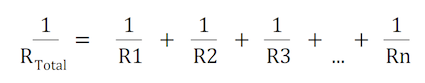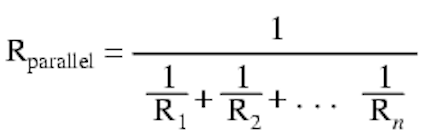### Area

• Area is the amount of space inside a flat shape.
• Area is measured in mm², cm² or m².
1. Rectangle area = length X width (breadth).
2. Triangle area = 1/2 X width X height.
3. Circle area = π(pi) X radius²
• π(pi) = 3.142

### Diameter

• The diameter, '∅', measures the size of a circle from one side to the other through the centre.
• The diameter is twice the radius.

## 3 Recap

### Practice Questions

1. You have a 12V battery and connect it to a 500Ω resistor, calculate the current flowing through the resistor. (3 marks)
2. Calculate the resistance needed if you have a 240V supply voltage and want to get 1A to flow through a component. (3 marks)
3. how many metres are in the following:
• 1km
• 1000mm
• 0.425km
4. If a circuit has the following resistors connected in parallel, calculate the total resistance for the following:
• 1000Ω and 500Ω
• 200Ω and 150Ω
• 500Ω and 500Ω
5. Does the E12 resistor series include a 4300Ω resistor?
6. Explain the different between surface-mounted and through hole soldering.
7. If a circuit has the following resistors connected in series, calculate the total resistance for the following:
• 1000Ω and 500Ω
• 200Ω and 150Ω
• 500Ω and 500Ω